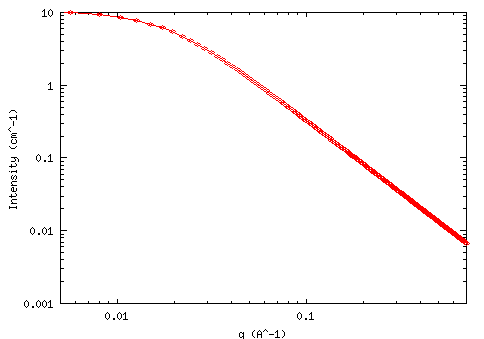MODEL

TwoHomopolymerRPA

AUTHOR/MODIFICATION

Alan Munter 08 JULY 1999

APPROVED FOR DISTRIBUTION

DESCRIPTION

Calculates the form factor, P(q), for a system of two homopolymers in the random phase approximation(RPA).

Resolution smeared version is also provided.

VARIABLES

Input Variables (default values):

Parameter Variable Value
0Degree of Polymerization A1000.0
1Degree of Polymerization B1000.0
2Volume Fraction of Polymer A0.2
3Volume of a Segment of Polymer A(cm3/mol)100.0
4Volume of a Segment of Polymer B(cm3/mol)100.0
5Contrast A-B(Å-2)2.0e-6
6Length of a Segment of Polymer A(Å)6.0
7Length of a Segment of Polymer B(Å)7.0
8Interaction between Polymers A and B0.0
9Incoherent Background (cm-1)0.0

USAGE NOTES

The function calculated is: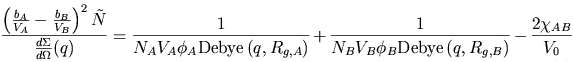where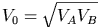,,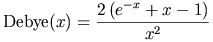,and with the following definitions: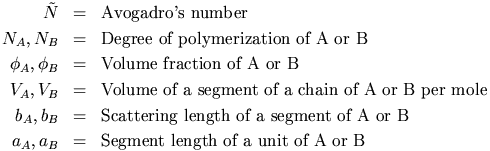The degree of polymerization in Parameter and Parameter is the number of base segments that are included in the polymer chains.

The Volume Fraction of Polymer B is calculated as (1 - Parameter).

Typical segment lengths are less than 10 Å for polymers like polystyrene.

If the interaction parameter is made too large it will result in unphysical results since the RPA is no longer applicable for strongly interacting polymers.

The returned value is in units of [cm-1] since it is scaled to the volume fractions of the polymers.

REFERENCE

TEST DATASET

This example dataset is produced by calculating the TwoHomopolymerRPA using 300 data points, qmin = 0.001 Å-1, qmax = 0.7 (Å-1) and the above default parameter values.